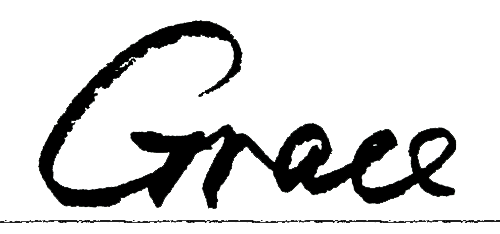# Python 标准库中 time 和 datetime 的区别与联系

Python 中提供了对时间日期的多种多样的处理方式，主要是在 timedatetime 这两个模块里。今天稍微梳理一下这两个模块在使用上的一些区别和联系。

### time

``````In : import time

In : time.time()
Out: 1414332433.345712
In : timestamp = time.time()

In : time.gmtime(timestamp)
Out: time.struct_time(tm_year=2014, tm_mon=10, tm_mday=26, tm_hour=14, tm_min=7, tm_sec=13, tm_wday=6, tm_yday=299, tm_isdst=0)

In : time.localtime(timestamp)
Out: time.struct_time(tm_year=2014, tm_mon=10, tm_mday=26, tm_hour=22, tm_min=7, tm_sec=13, tm_wday=6, tm_yday=299, tm_isdst=0)
In : struct_time = time.localtime(timestamp)

In : time.ctime(timestamp)
Out: 'Sun Oct 26 22:07:13 2014'

In : time.asctime(struct_time)
Out: 'Sun Oct 26 22:07:13 2014'

In : time.mktime(struct_time)
Out: 1414332433.0

In : time.strftime("%a, %d %b %Y %H:%M:%S +0000", struct_time)
Out: 'Sun, 26 Oct 2014 22:07:13 +0000'

In : time.strptime("30 Nov 00", "%d %b %y")
Out: time.struct_time(tm_year=2000, tm_mon=11, tm_mday=30, tm_hour=0, tm_min=0, tm_sec=0, tm_wday=3, tm_yday=335, tm_isdst=-1)

``````

### datetime

datetime 比 time 高级了不少，可以理解为 datetime 基于 time 进行了封装，提供了更多实用的函数。在datetime 模块中包含了几个类，具体关系如下:

``````object
timedelta     # 主要用于计算时间跨度
tzinfo        # 时区相关
time          # 只关注时间
date          # 只关注日期
datetime  # 同时有时间和日期
``````

``````datetime.year
datetime.month
datetime.day
datetime.hour
datetime.minute
datetime.second
datetime.microsecond
datetime.tzinfo

datetime.date() # 返回 date 对象
datetime.time() # 返回 time 对象
datetime.replace(name=value) # 前面所述各项属性是 read-only 的，需要此方法才可更改
datetime.timetuple() # 返回time.struct_time 对象
dattime.strftime(format) # 按照 format 进行格式化输出
...
``````

``````datetime.today()  # 当前时间，localtime
datetime.now([tz]) # 当前时间默认 localtime
datetime.utcnow()  # UTC 时间
datetime.fromtimestamp(timestamp[, tz]) # 由 Unix Timestamp 构建对象
datetime.strptime(date_string, format)  # 给定时间格式解析字符串
...
``````

``````In : import datetime
In : time_now = datetime.datetime.now()
In : time_now
Out: datetime.datetime(2014, 10, 27, 21, 46, 16, 657523)

In : delta1 = datetime.timedelta(hours=25)
In : print(time_now + delta1)
2014-10-28 22:46:16.657523

In : print(time_now - delta1)
2014-10-26 20:46:16.657523
``````

### 小结

blog comments powered by Disqus